##1. Involutive homography on a conic

Given a conic c, an involutive homography of c is a bijective map H of c onto c, which immitates the diametral map of a circle. Namely there is a point F, such that for every point X on the conic H(X) is the other intersection point of the conic with line FX.
F is called the Fregier point of the involutive homography. The polar line L of F with respect to the conic is called the homography axis of the involutive homography.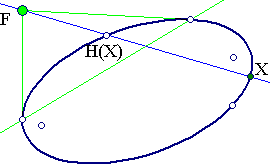##2. Other aspects

Obviously, given the conic (c), an involutive homography is defined uniquely by giving a point (F) or a line (L). This because F defines L as its polar and L, in turn, defines F as its pole with respect to the conic. The involutive homography H of the conic coinsides then with the restriction on the conic (c) of the harmonic perspectivity, with respect to the pair (F,L).
Another aspect is through the use of projective coordinates along the conic and more precisely of a good parametrization S (see GoodParametrization.html ) on (c). Using this the map H is represented through H' = S*H*S-1 and the involution requirement is equivalent with the propery of H' to by a line involution i.e. of the form x'=(ax+b)/(cx-a)).

##3. Invariant families of conics

Consider a conic c a point F and its polar e with respect to that conic. c and e (considered as a degenerate double-line conic) generate a family of conics {c'} (in the construction below controlled by the point P). Line e is the polar of F for all these conics. Using F and its polar one can define a global (i.e. defined on the whole projective plane) involutive homography (projectivity) H, which on each member c' of the family restricts to an involutive homography with Fregier point F.
The obvious definition is to associate to each point X of c' the other intersection point X' of XF with c'. If D is the intersection point of FX with e then, by the definition of the polar, the four points will make a harmonic division: (X,X',F,D) = -1. Projectivities of this kind are called harmonic perspectivities.
Since their definition depends only on F and line e, we expect to find many families of conics, like {c'} invariant under this projectivity. Indeed, every conic c'' having (F, e) as pole-polar respectively, generates together with e a family of conics invariant under this projectivity (see Harmonic_Perspectivity.html for a discussion).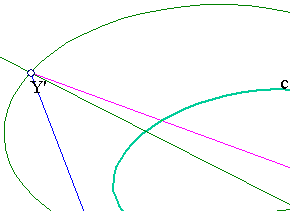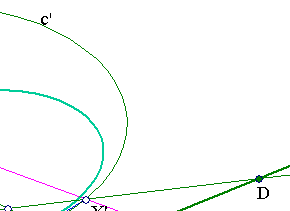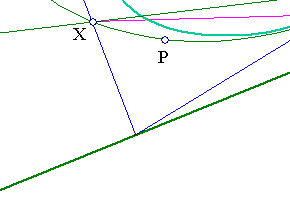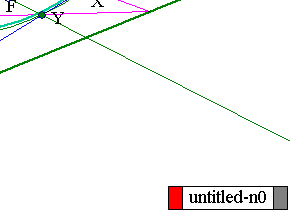##4. Particular cases

 When e is the line at infinity, F being then the center of the conic (must have one). The corresponding involution being the symmetry on the center of the conic.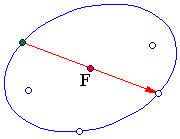When e passes through the center, F being then at infinity the involution being then a conjugate diameter involution (see Conjugate_Diameter_Involution.html .), by which each point X maps to X', such that XX' is parallel to e, hence its middle MX is on the conjugate diameter e' of e.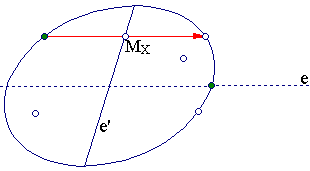The so-called Fregier involution associated with a particular point W of the conic (c). In this, every point X of the conic other than W maps to X', such that the angle XWX' is a right one.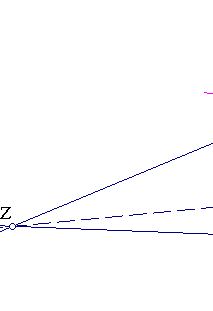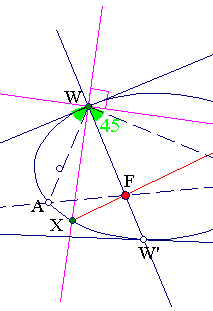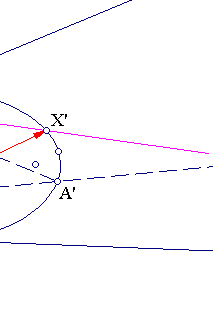Once shown that this map is indeed an involution (see Fregier.html ), one can easily show that the Fregier point F of the involution is on the normal at W. Indeed the involution interchanges points {W,W'}, where W' the other intersection point with the normal at W. Take also the bisectors {WA, WA'} of the right angle at W, {A,A'} being their other intersection points with the conic. F coincides with the intersection point of lines {AA', WW'}.